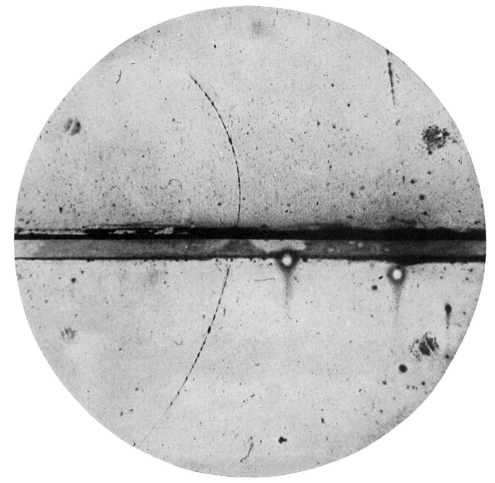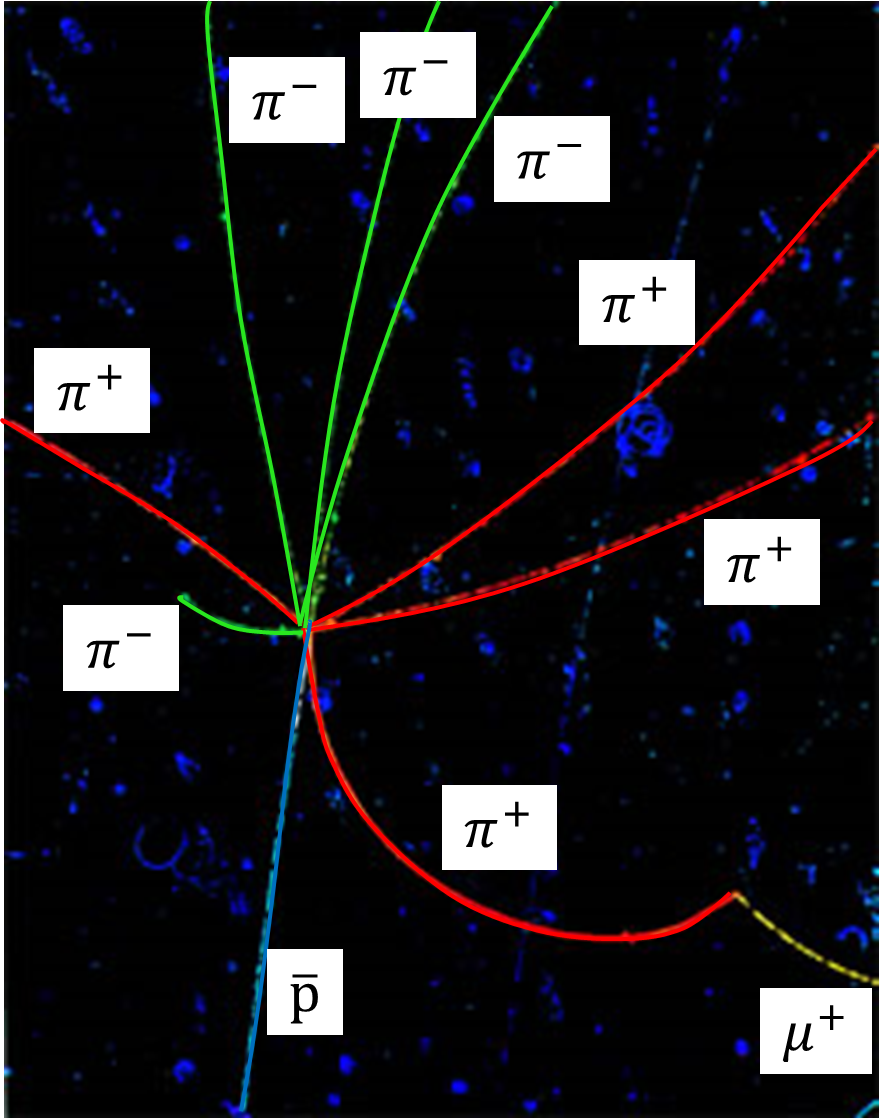## Section58.1Antiparticles

We will start survey of fundamental particles with the story of the prediction and discovery of positrons, which are antiparticle of electron. Positron has same mass as the electron but it has exactly opposite charge.

Quantum mechanics enjoyed a number of successes immediately after it was proposed by Schr\"odinger in 1925. However, it was not consistent with Einstein's theory of relativity. That is, one could not make quantum predictions for the motion of particles at speeds close to the speed of light. In 1928 the British physicist Paul A. M. Dirac derived a relativistic wave equation for which he was awarded the Nobel Prize in Physics in 1933. Dirac showed that his equation described the properties of electrons well explaining even the gyromagnetic factor 2 for the electron.

There was one serious problem with Dirac theory: the solution of the Dirac equation had both positive energy and negative energy solutions and there was no reason to throw away the negative energy solutions as unphysical. However, suppose you have an electron in a positive energy state with energy $E_a\text{,}$ and a negative energy state $E_{b}$ is unoccupied, then, based on Bohr's model, the electron will make a transition from state $a$ to state $b$ and a photon of energy $E_a-E_b$ will be emitted. $hf = E_a - E_b.$ Since states with all negative energy values were allowed in the Dirac equation, this process would continue ad infinitum. That is, the Dirac equation could not explain why electrons are stable. To avoid this instability in the solution, Dirac suggested that somehow all the negative energy solutions were occupied, the so-called negative energy sea. Now, if a state is occupied, then Pauli's exclusion principle will prohibit another electron from accessing that state. Now, if an electron in the negative energy state is excited, then it will leave a hole in the negative energy sea, which would be similar to the hole in the valence band of metals and semiconductors we have discussed in the context of solid-state physics.

Since a hole in the negative sea is simply an unoccupied negative-energy state it will have a positive energy because it is a place where there is a shortage of negative energy. That is, a hole in the negative sea is just like a regular particle with positive energy. When Dirac studied the motion of these holes in an electromagnetic field he found that the hole will act like an electron with the opposite charge of an electron and mass equal to the mass of the electron. $m_{\textrm{hole}} = m_e,\ \ q_{\textrm{hole}} = + e,\ \ \textrm{spin}_{\textrm{hole}} = \textrm{spin}_{e} = \frac{1}{2}.$ C. D. Anderson at Caltech, USA, discovered a particle matching these properties in 1932 in photographs of the particle tracks of cosmic rays in a cloud chamber. Figure 58.1.1 shows a photograph taken by Anderson of a particle bending in a magnetic field. The analysis of the track showed that the particle had the mass of an electron but had exactly opposite charge.Figure 58.1.1. Positron tracks in a Bubble chamber in a magnetic field by C. D. Anderson, Physical Reviews, Vol. 33, pp 491-493 (1933). the horizontal part is a lead bar of thickness 6 mm. The photograph shows a positron of energy 63 MeV (upper) piercing through the 6mm lead plate and emerging as a 24 MeV positron.

Out of about 1300 photographs taken by Anderson 15 tracks had these particles. Anderson coined the term positron for this particle. This was the first time physicists had discovered an antiparticle for which Anderson was awarded Nobel Prize for Physics in 1936. It is notable that there was evidence for this particle in earlier work by others that had gone unnoticed or misinterpreted, e.g. in the work of Chung-Yao Chao, a graduate student also at Caltech, who had seen some peculiar tracks and didn't follow up on his discovery.

When a positron comes in contact with an electron they annihilate each other producing two photons.

\begin{equation*} e^{+} + e^{-} \rightarrow \gamma + \gamma. \end{equation*}

The time reversal symmetry of electromagnetism, meaning equations of electromagnetism being symmetric in $t \leftrightarrow -t$ substitution, suggests that the reaction could proceed backwards also. That is, two photons of sufficient energy could strike and produce an electron-positron pair. Rather than perform exactly the time reversal of pair annihilation, we send a photon of sufficiently high energy on a nuclear target. We find that a pair production of electron and positron takes place,

\begin{equation*} \gamma + M \rightarrow e^{+} + e^{-} + N, \end{equation*}

where $M$ is the target nucleus state and $N$ is the product nucleus state if energy of the photon is at least twice the rest energy of an electron.

\begin{equation*} E_{\textrm{min}}[\textrm{photon}] = 2 m_e c^2 = 2\times 0.511\:\textrm{MeV} = 1.022\:\textrm{MeV}. \end{equation*}

### Subsection58.1.1Other Antiparticles

After the discovery of anti-electron (positron) it was generally believed that every particle has its anti-particle. However, anti-proton was not discovered till 1955 by Emilio Segrè, Owen Chamberlain, Clyde Wiegand and Thomas Ypsilantis at Lawrence Berkeley Laboratory, California, for which Emilio Segrè and Owen Chamberlain received the 1959 Nobel Prize in Physics. The team under Emilio Segrè and Owen Chamberlain accelerated protons to 6.3 GeV and struck stationary copper block target. They needed at least two times the rest energy of proton to generate the proton-antiproton pair.

\begin{equation*} \textrm{p} + M \rightarrow \textrm{p} + \bar{\textrm{p}} + N, \end{equation*}

where $M$ is the target nucleus and $N$ is the product nucleus. The antiproton was detected by measuring its speed and momentum, and characteristic star pattern on an emulsion plate when antoproton annihilated a proton of the medium. One such annihilation is shown in Figure 58.1.2. From the curvatures of the tracks it was possible to identify various particles in the photograph. The antiproton enters the plate from lower bottom and annihilates a proton in some atom of the emusion material, forming a number of fragments. In this figure, they identified four negative pion and four positive pions.Figure 58.1.2. Discovery of antiproton. The antiproton enters from the lower left. The annihilation of the antiproton with a proton of the medium releases fragments expected from proton-antiproton annihilation. Courtesy of Lawrence Berkeley Laboratory.

Physicists now believe that every particle has an antiparticle that has the same mass, same spin, and opposite charge. There are some exceptions to this general rule - for instance, photon is anti-particle of itself, i.e., there is no separate anti-photon particle. We denote the antiparticle of a particle by placing a bar over the symbol of the particle. For instance, the anti-particle of proton p is denoted by $\bar{\textrm{p}}\text{,}$ the anti-particle of neutron n by $\bar{\textrm{n}}\text{,}$ anti-neutrino by $\bar\nu\text{,}$ and so on. The anti-particle of electron is sometimes not indicated this way, instead we use $e^{+}$ for the positron and $e^{-}$ for the electron.

The particle-antiparticle symmetry electromagnetic interaction suggests that if we replace all electrons, protons, and neutrons in an atom by their antiparticles we should be able to make anti-atom. An anti-hydrogen $\bar{\textrm{H}}$ was first synthesized at the CERN Antiproton Decelerator (AD) in 2002. Of course, such a hydrogen atom will annihilate a regular hydrogen atom if they come in contact. By putting together anti-atoms we can get anti-molecules, and in this way, we can build materials entirely from anti-matter. A mystery of the universe is that in our part of the universe, we have mostly matter. Finding an explanation of this fact is a hot topic of research. Among the explanations is an explanation based on the discovery that the decay of certain particles produce very a little more electron than positron. From this observation, it has been suggested that, in the early universe, within first minute or so of the Big Bang, more matter was formed than antimatter. Subsequently, matter and antimatter annihilated each other leaving the excess matter that we see today.

TODO:

\​begin{exercise} List five particles with their antiparticles. \end{exercise}

\​begin{exercise} The antiparticle of a proton is an antiproton. (a) List two properties of antiproton that are same as those of a proton. (b) List two properties of antiproton that are different from those of a proton. \end{exercise}

\​begin{exercise} (a) What is the antiparticle of a photon? (b) Are there other particles that have the same type of relation between particle and antiparticle? Give at least two other examples. \end{exercise}

\​begin{exercise} A positron beam of energy 2.0 MeV collides with a silver atom at rest. Two photons come out when an electron in the silver atom annihilates the positron. Assuming that the energy of the silver atom is not changed appreciably, except the loss of an electron, and that the speed of the electron in the silver atom can be ignored, what are the frequencies of the photons that come out? \end{exercise}

\​begin{exercise} In the text we have discussed the production of antiproton by a beam of protons striking a proton-rich target. $\textrm{p} + \textrm{p} \rightarrow \textrm{p} + \textrm{p} + \textrm{p} + \bar{\textrm{p}}.$ In addition to this reaction the reaction prducing antiproton also occurs. $\textrm{p} + \textrm{p} \rightarrow \textrm{p} + \textrm{p} + \textrm{n} + \bar{\textrm{n}}.$ \end{exercise}